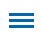A key component of sixth-grade mathematical instruction involves the use of ratio and rate. This includes extending equivalent ratio and rates as well as analyzing simple scale drawings.

Editor's Note: This blog is part of a series of posts from former math specialist Scott Loomis on the real-world math templates he has created for Wixie and how you can use and modify them for your own awesome performance tasks. You can read the backstory on his experience and expertise below.

The Sky-High Skyscrapers template in Wixie requires students to measure the length of the image in centimeters and, using a key, convert the distance to meters. This task is completed five times before students generate an image of their own specific to a structure in their city, state, or geographic region.Instructional Concept: Instructional Concept: Ratio & Proportional Relationships

Template Location in Wixie: Curriculum> Math > Algebra > Ratio and Rate

Estimated Completion Time: 15-25 minutes

Wixie Sky-High Skyscrapers Template

## Math Standards and Learning Goals - 6th Grade

Common Core State Standards - Mathematics -Ratio & Proportional Relationships
CCSS.MATH.CONTENT.6.RP.A.3 Use ratio and rate reasoning to solve real-world and mathematical problems, e.g., by reasoning about tables of equivalent ratios, tape diagrams, double number line diagrams, or equations. (D) Use ratio reasoning to convert measurement units; manipulate and transform units appropriately when multiplying or dividing quantities.

Virginia SOL - Mathematics - Patterns, Functions, and Algebra
6.12.a The student will represent a proportional relationship between two quantities, including those arising from practical situations.

Texas TEKS - Mathematics - Proportionality
6.4.B The student applies mathematical process standards to develop an understanding of proportional relationships in problem situations. The student is expected to apply qualitative and quantitative reasoning to solve prediction and comparison of real-world problems involving ratios and rates.

## Wixie Skills Review:

The manipulation of a fix-shaped ruler is required to measure the length of each skyscraper image. A review of proper use of the ruler -- 'zero' does not start at the very end of the ruler, but at the marked line -- is encouraged. The metric ruler cannot be resized unless the student (likely intentionally) explores options to disable this setting. If the ruler is resized, the easiest fix would be to copy and paste a ruler from pages 2, 3, 4, or 6 of the template.

Students are also required to record their measurements in the provided tables and can do so with the pencil tool or by adding a text object. Students should be assessed on both their measuring skills and conversation (multiplication) calculation.

Page 5 requires students to record an explanation of the perceived accuracy of their conversation compared to the actual height of the given buildings. Responses may vary, but supporting their mathematical thinking is a critical component when assessing this part of the template.

The final page, page 6, of the activity requires students to draw a scale model of an actual building from their city, state, or even region. It is encouraged that students be given minimum time to research -- the 'just pick one already' strategy may prove to be of value -- before beginning the illustration. Encourage students to use the line or shape options available within the Paint a Shape draw tools.

## Making your own custom templates in Wixie

Wixie is a powerful canvas for elementary math learning. Wixie makes it easy to combine typed and drawn numbers with math manipulatives and audio explanations of mathematical thinking.

With limited classroom time, you can create or customize Wixie templates to guide student math practice and independent work to meet specific math goals in your classroom.

Topics: Wixie, collaboration, real world of math